Next: The Mathematical Details Up: Some Consequences Previous: The Cosmological Constant

### Spatial Curvature

We have emphasized that gravity is due not just to the curvature of space, but of spacetime. In our verbal formulation of Einstein's equation, this shows up in the fact that we consider particles moving forwards in time and study how their paths deviate in the space directions. However, Einstein's equation also gives information about the curvature of space. To illustrate this, it is easiest to consider not an expanding universe but a static one.

When Einstein first tried to use general relativity to construct a model of the entire universe, he assumed that the universe must be static -- although he is said to have later described this as his greatest blunder''. As we did in the previous section, Einstein considered a universe containing ordinary matter with density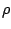, no pressure, and a cosmological constant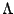. Such a universe can be static -- the galaxies can remain at rest with respect to each other -- only if the right-hand side of equation (4) is zero. In such a universe, the cosmological constant and the density must be carefully `tuned' so that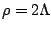. It is tempting to conclude that spacetime in this model is just the good old flat Minkowski spacetime of special relativity. In other words, one might guess that there are no gravitational effects at all. After all, the right-hand side of Einstein's equation was tuned to be zero. This would be a mistake, however. It is instructive to see why.

Remember that equation (2) contains all the information in Einstein's equation only if we consider all possible small balls. In all of the cosmological applications so far, we have applied the equation only to balls whose centers were at rest with respect to the local matter. It turns out that only for such balls is the right-hand side of equation (2) zero in the Einstein static universe.

To see this, consider a small ball of test particles, initially at rest relative to each other, that is moving with respect to the matter in the universe. In the local rest frame of such a ball, the right-hand side of equation (2) is nonzero. For one thing, the pressure due to the matter no longer vanishes. Remember that pressure is the flux of momentum. In the frame of our moving sphere, matter is flowing by. Also, the energy density goes up, both because the matter has kinetic energy in this frame and because of Lorentz contraction. The end result, as the reader can verify, is that the right-hand side of equation (2) is negative for such a moving sphere. In short, although a stationary ball of test particles remains unchanged in the Einstein static universe, our moving ball shrinks!

This has a nice geometric interpretation: the geometry in this model has spatial curvature. As we noted in section 2, on a positively curved surface such as a sphere, initially parallel lines converge towards one another. The same thing happens in the three-dimensional space of the Einstein static universe. In fact, the geometry of space in this model is that of a 3-sphere. This picture illustrates what happens: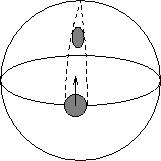One dimension is suppressed in this picture, so the two-dimensional spherical surface shown represents the three-dimensional universe. The small shaded circle on the surface represents our tiny sphere of test particles, which starts at the equator and moves north. The sides of the sphere approach each other along the dashed geodesics, so the sphere shrinks in the transverse direction, although its diameter in the direction of motion does not change.

As an exercise, the reader who wants to test his understanding can fill in the mathematical details in this picture and determine the radius of the Einstein static universe in terms of the density. Here are step-by-step instructions:

• Imagine an observer moving at speed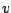through a cloud of stationary particles of density. Use special relativity to determine the energy density and pressure in the observer's rest frame. Assume for simplicity that the observer is moving fairly slowly, so keep only the lowest-order nonvanishing term in a power series in.

• Apply equation (2) to a sphere in this frame, including the contribution due to the cosmological constant (which is the same in all reference frames). You should find that the volume of the sphere decreases with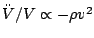to leading order in.

• Suppose that space in this universe has the geometry of a large 3-sphere of radius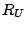. Show that the radii in the directions transverse to the motion start to shrink at a rate given by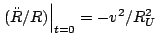. (If, like most people, you are better at visualizing 2-spheres than 3-spheres, do this step by considering a small circle moving on a 2-sphere, as shown above, rather than a small sphere moving on a 3-sphere. The result is the same.)

• Since our little sphere is shrinking in two dimensions, its volume changes at a rate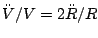. Use Einstein's equation to relate the radiusof the universe to the density.

The final answer is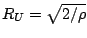. As you can confirm in standard textbooks, this is correct.

Spatial curvature like this shows up in the expanding cosmological models described earlier in this section as well. In principle, the curvature radius can be found from our formulation of Einstein's equation by similar reasoning in these expanding models. In fact, however, such a calculation is extremely messy. Here the apparatus of tensor calculus comes to our rescue.

Next: The Mathematical Details Up: Some Consequences Previous: The Cosmological Constant

© 2006 John Baez and Emory Bunn